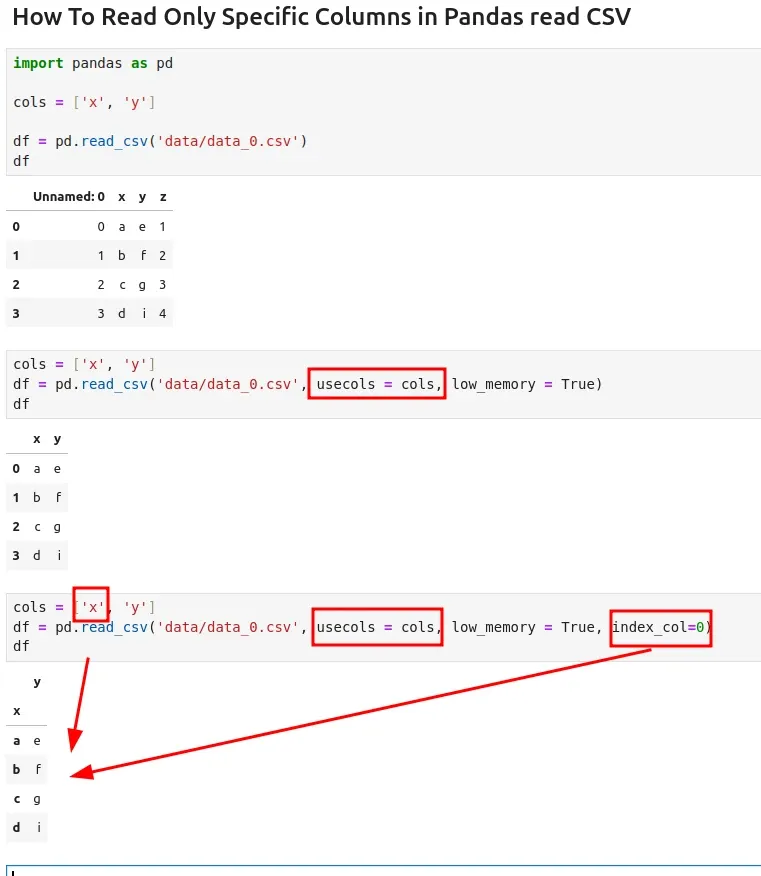To read only specific columns from CSV file using Pandas read_csv method we need to use parameter `usecols=fields`

## Steps to read specific columns from CSV file

• Import pandas
• Define columns to be read
• `usecols=fields` - to list columns to be read
• Subset of columns to select, denoted either by column labels or column indices.
• `low_memory = True` - reading in chunks
• Internally process the file in chunks, resulting in lower memory use while parsing, but possibly mixed type inference.
• `index_col` - to identify column X as index
• Column(s) to use as row label(s), denoted either by column labels or column indices.
• `names` and `header` to override the column names.

## Data

Suppose we have the following CSV file which we like to read with Pandas. We want to read only single column from this CSV file into DataFrame:

``````,x,y,z
0,a,e,1
1,b,f,2
2,c,g,3
3,d,i,4
``````
x y z
0 a e 1
1 b f 2
2 c g 3
3 d i 4

## Example

``````import pandas as pd
cols = ['x', 'y']
df = pd.read_csv('data/data_0.csv', usecols = cols, low_memory = True)
``````

## Output